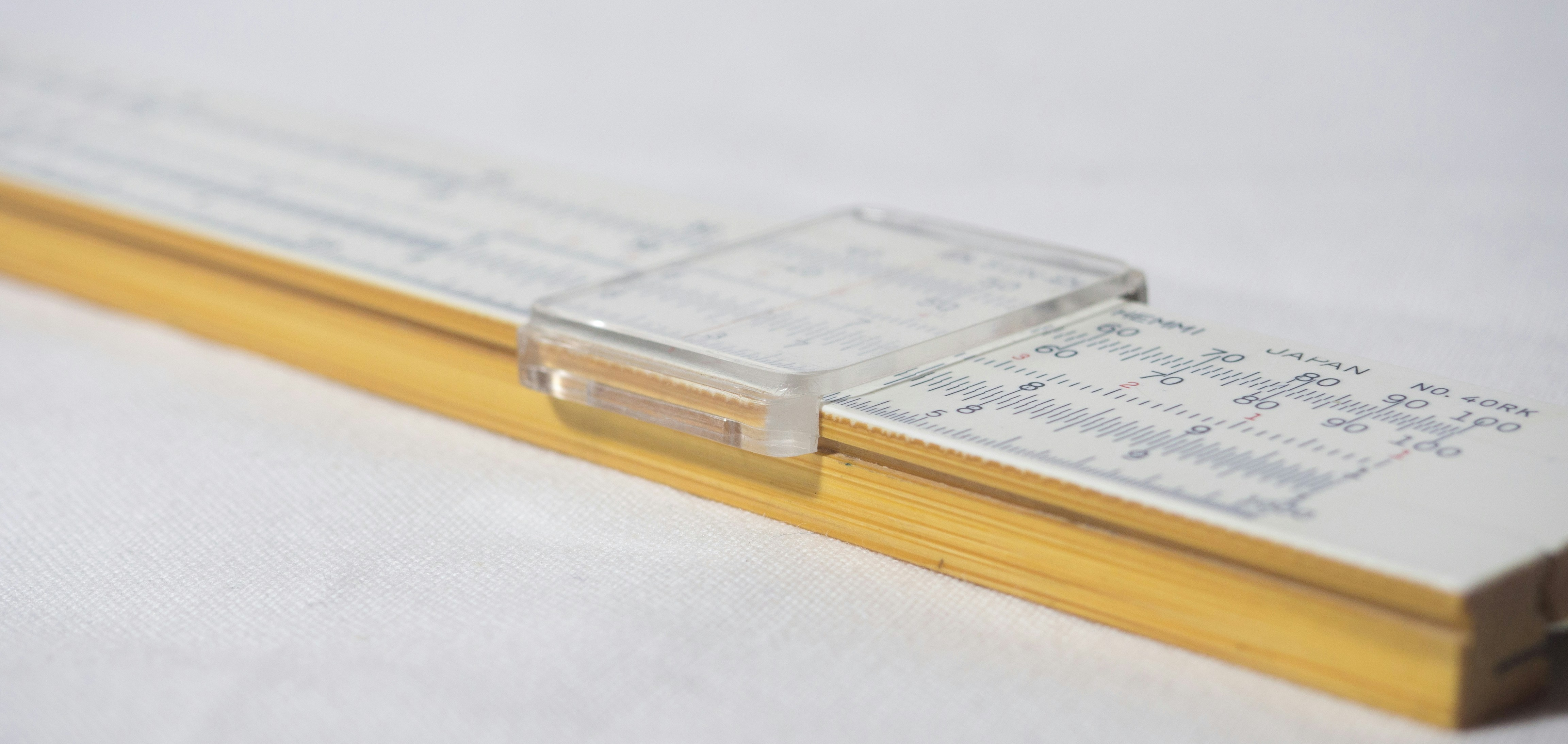😚

# 线性代数复习

Tags
GAMES101
Published
Published May 11, 2022
Author

### 点乘 Dot (scalar) Product

In 2D
In 3D

#### Dot Product in Graphics

• Find angle between two vectors (e.g. cosine of angle between light source and surface)
• Finding projection of one vector on another

### 向量叉乘 Cross (vector) Product

• Cross product is orthogonal to two initial vectors
• Direction determined by right-hand rule
• Useful in constructing coordinate systems (later)

#### Cross Product in Graphics

1. 判断左和右：叉乘可以判断一个向量是否在另一个向量左侧还是右侧（二维平面），右手坐标系下，如果向量 a 叉乘向量 b 结果 z 为正，说明 b 在 a 的左侧。
1. 判断内和外：判断一个点在不在中间，可以顺时针看 ABC，对向量 AB 和 AP 叉乘，BC 和 BP，CA 和 CP，如果结果都同为正或同为负，说明点 P 在三个向量的同一侧，即使是逆时针 ACB 这么算向量叉乘，如果点 P 在三角形中，三个叉乘结果也应该是相同符号的（同是正或同是负）。

### Orthonormal Bases / Coordinate Frames

• Important for representing points, positions, locations
• Often, many sets of coordinate systems
• Global, local, world, model, parts of model (head, hands, …)
• Critical issue is transforming between these systems/bases
• A topic for next week

### Matrices 矩阵

• Magical 2D arrays that haunt in every CS course
• In Graphics, pervasively used to represent transformations
• Translation, rotation, shear, scale (more details in the next lecture)

#### What is a matrix

Array of numbers (m × n = m rows, n columns)
Addition and multiplication by a scalar are trivial: element by element

#### Matrix-Matrix Multiplication 矩阵的乘积

#(number of) columns in A must = # rows in B
(M x N) (N x P) = (M x P)

Element in the product is the dot product of row i from A and column j from B

#### Properties

• Non-commutative 没有任何交换律（某些情况可以） (AB and BA are diﬀerent in general)
• Associative and distributive 有结合律和分配律

#### Matrix-Vector Multiplication

• Treat vector as a column matrix (m×1)
• Key for transforming points (next lecture)

• Oﬃcial spoiler: 2D reﬂection about y-axis

#### Transpose of a Matrix

Switch rows and columns (ij -> ji)

Dot product?

Cross product?